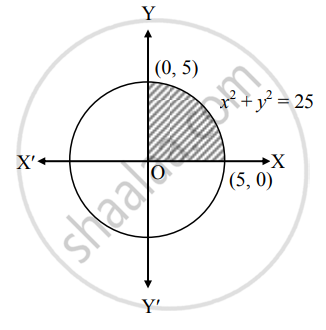# Find the area of sector bounded by the circle x2 + y2 = 25, in the first quadrant - Mathematics and Statistics

Sum

Find the area of sector bounded by the circle x2 + y2 = 25, in the first quadrant−

#### Solution

Given equation of the circle is x2 + y2 = 25

∴ y2 = 25 − x2

∴ y = +-  sqrt(25 - x^2)

∴ y = sqrt(25 - x^2)    .....[∵ In first quadrant, y > 0]∴ Required area = int_0^5 y  "d"x

= int_0^5 sqrt(25 - x^2)  "d"x

= [x/2 sqrt(25 - x^2) + 25/2 sin^-1 (x/5)]_0^5

= 0 + 25/2 sin^-1 (1) - 0

= 25/2 (pi/2)

= (25pi)/4 sq.units

Concept: Area Between Two Curves
Is there an error in this question or solution?